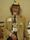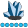## Error: Expression using equals (=) has components that are of different data types)

Hi there,

I get the above error message (Error: Expression using equals (=) has components that are of different data types) upon running the following code:

%MACRO eventsum(period=);
proc sql;
create table disease_standard as
select year, age_group, sex, disease
from dataset_name
where disease=1 and year=&period;
quit;
run;

%MEND eventsum;
%eventsum(period=2013)
%eventsum(period=2014)
%eventsum(period=2015)
%eventsum(period=2016)
%eventsum(period=2017)

I'm not sure what the error message means, and how to go about fixing it. Any help?

Thanks

5 REPLIES 5

## Re: Error: Expression using equals (=) has components that are of different data types)

You miss semicolons on all lines executing the macro. It should be:

``````%eventsum(period=2013);
%eventsum(period=2014);
%eventsum(period=2015);
%eventsum(period=2016);
%eventsum(period=2017);``````

Alternatively, if have semicolons and this is just typo on post,

then probably PERIOD is defined as charcter and you have to code execution like:

``````%eventsum(period='2013');
%eventsum(period='2014');
%eventsum(period='2015');
%eventsum(period='2016');
%eventsum(period='2017');``````

## Re: Error: Expression using equals (=) has components that are of different data types)

Thanks for the response. When I make the suggested modifications to the SAS
code (added single quotes and semicolons), I get the following error codes:

*ERROR 22-322: Syntax error, expecting one of the following: (, AS, LIKE.*

*ERROR 200-322: The symbol is not recognized and will be ignored.*

Not sure why I continue to get error messages...

## Re: Error: Expression using equals (=) has components that are of different data types)

Post your new code and log.

Ideally, a proc contents on your data set as well, unless you're sure you've corrected the type issue already.

## Re: Error: Expression using equals (=) has components that are of different data types)

Check your variable types for the two variables in your WHERE clause. If either are character they require quotes around the values.

``where disease='1' and year=&period``

or

``where disease=1 and year="&period"``

Or possibly both combined.Ron_MacroMaven
Lapis Lazuli | Level 10

## Re: Error: Expression using equals (=) has components that are of different data types)

proc sql; describe table dataset_name;

quit;

the log will show you the data structure;

use quotes accordingly

as noted by an earlier responder.

Ron Fehd  data structure!

Discussion stats
• 5 replies
• 3549 views
• 1 like
• 4 in conversation